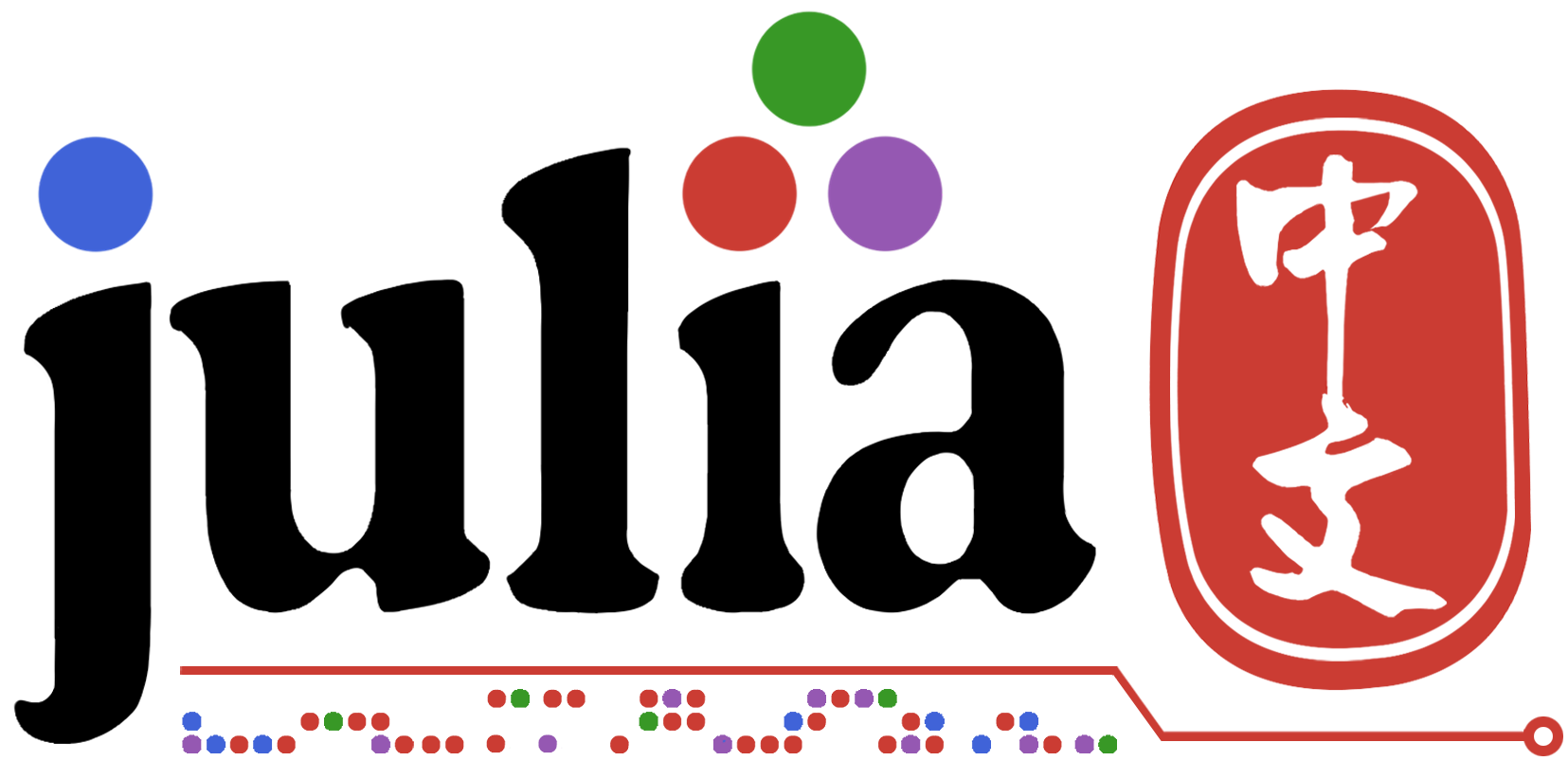# 直方图绘制，纵轴为密度

``````help?> histogram
search: histogram histogram! histogram2d histogram2d!

histogram(x)
histogram!(x)

Plot a histogram.

Arguments
≡≡≡≡≡≡≡≡≡≡≡

•  x: AbstractVector of values to be binned

•  bins: Integer, NTuple{2,Integer}, AbstractVector or Symbol. Default is :auto (the Freedman-Diaconis rule). For histogram-types, defines the
approximate number of bins to aim for, or the auto-binning algorithm to use (:sturges, :sqrt, :rice, :scott or :fd). For fine-grained control
pass a Vector of break values, e.g. range(minimum(x), stop = maximum(x), length = 25)

•  weights: Vector of weights for the values in x, for weighted bin counts

•  normalize: Bool or Symbol. Histogram normalization mode. Possible values are: false/:none (no normalization, default), true/:pdf (normalize
to a discrete Probability Density Function, where the total area of the bins is 1), :probability (bin heights sum to 1) and :density (the
area of each bin, rather than the height, is equal to the counts - useful for uneven bin sizes).

•  bar_position: Symbol. Choose from :overlay (default), :stack. (warning: May not be implemented fully)

•  bar_width: nothing or Number. Width of bars in data coordinates. When nothing, chooses based on x (or y when orientation = :h).

•  bar_edges: Bool. Align bars to edges (true), or centers (the default)?

•  orientation: Symbol. Horizontal or vertical orientation for bar types. Values :h, :hor, :horizontal correspond to horizontal (sideways,
anchored to y-axis), and :v, :vert, and :vertical correspond to vertical (the default).

Example
≡≡≡≡≡≡≡≡≡

julia> histogram([1,2,1,1,4,3,8],bins=0:8)
julia> histogram([1,2,1,1,4,3,8],bins=0:8,weights=weights([4,7,3,9,12,2,6]))
``````

``````histogram(A,normalize=:probability)
``````